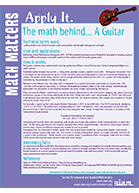Search IntMath
Close

# Math Matters, Apply It

By Murray Bourne, 08 May 2007

There are some nice PDF posters on some of the applications of mathematics in Math Matters, Apply It!, by The Society for Industrial and Applied Mathematics (SIAM).

According to their site:

"Math Matters, Apply It!" is an applied mathematics awareness campaign, created for anyone who wants to know more about the mathematics behind everyday life and the technologies we encounter.

The posters cover topics like

1. The Math behind Digital Animation
2. The Math behind Using DNA
3. The Math behind GuitarsThe posters are similar to the ones by the American Mathematical Society that I wrote about before in Mathematical Moments.

Both are worth a look.

Be the first to comment below.

### Comment Preview

HTML: You can use simple tags like <b>, <a href="...">, etc.

To enter math, you can can either:

1. Use simple calculator-like input in the following format (surround your math in backticks, or qq on tablet or phone):
a^2 = sqrt(b^2 + c^2)
(See more on ASCIIMath syntax); or
2. Use simple LaTeX in the following format. Surround your math with $$ and $$.
$$\int g dx = \sqrt{\frac{a}{b}}$$
(This is standard simple LaTeX.)

NOTE: You can mix both types of math entry in your comment.